# Surface Tension Worksheet

In this page we have Surface Tension Worksheet . Hope you like them and do not forget to like , social share and comment at the end of the page.

# Derivations and definition based problems

Question 1 Define Surface tension and explain surface tension on the basis of molecular theory.
Question 2 Define Surface energy. Prove it is numerically equal to surface tension.
Question 3 Show that a pressure difference exists between two sides of curved liquid surface.
Question 4 Derive an expression for excess pressure
(a) Inside a liquid drop
(b) Inside a soap bubble.
Question 5 What do you understand by the term capillarity? Give some examples of capillarity from daily life.
Question 6 Derive an expression for the rise of liquid in capillary tube and show that the height of the liquid column supported is inversely proportional to the radius of the tube.

# Numerical based problems

Question 1 Calculate the work done in blowing in a soap bubble from radius 2 cm to 3 cm. The surface tension of the soap solution is 30 dyne/cm. (Answer:- 3770.4 erg)
Question 2 The liquid drop of diameter D breaks up into 27 tiny drops. Find the resulting change in energy. Take surface tension of liquid as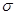.  (Answer:-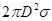)
Question 3 If 500 erg of work is done in blowing a soap bubble of a radius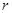, what additional work is required to be done to blow it to a radius equal to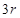? (Answer:-  4000 erg)
Question 4 Soapy water drips from capillary. When the drop breaks away , the diameter of its neck is 1 mm. The mass of the drop is 0.0129 g. Find the surface tension of the soapy water. (Answer:-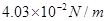)
Question 5 If the number of little droplets of water of surface tension, all of the radius, combine to form a single drop of radius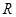and the energy released is converted into kinetic energy, find the velocity acquired by the bigger drop.
Question 6 What should be the pressure inside a small air bubble of 0.1 mm radius, situated just below the surface?  Surface tension of water =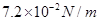and
atmospheric pressure =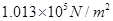Question 7 Two soap bubbles have radii in ratio 2:3. Compare the excess pressure of liquid inside these bubbles. Also compare the works done in blowing these bubbles.
Question 8 Water rises in a capillary tube to a height of 2.0 cm. In another capillary tube whose radius is one-third of it, how much the water will rise? (Answer :-  6.0 cm )
Question 9 A small hollow sphere having a small hole in it is immersed into water to a depth of 20 cm before any water penetrates into it. If the surface tension of water is 73 dynes cm–1, find the radius of the hole. (Answer:-  0.0075 cm)
Question 10 The maximum force, in addition to the weight required to pull a wire 5 cm long from the surface of water at 20°C is 728 dynes. Calculate the surface tension of water.  (Answer:- 72.8 dynes/cm)